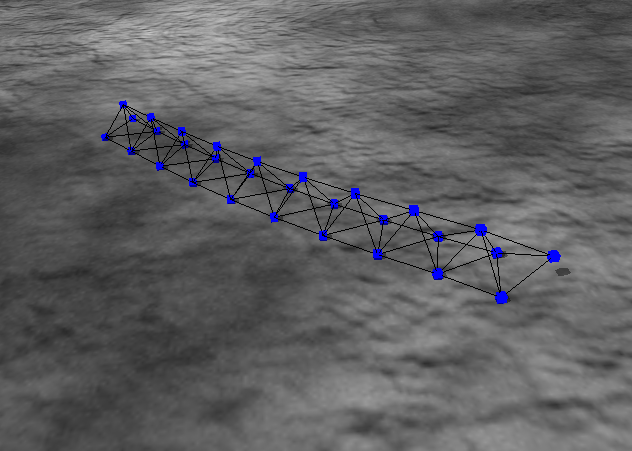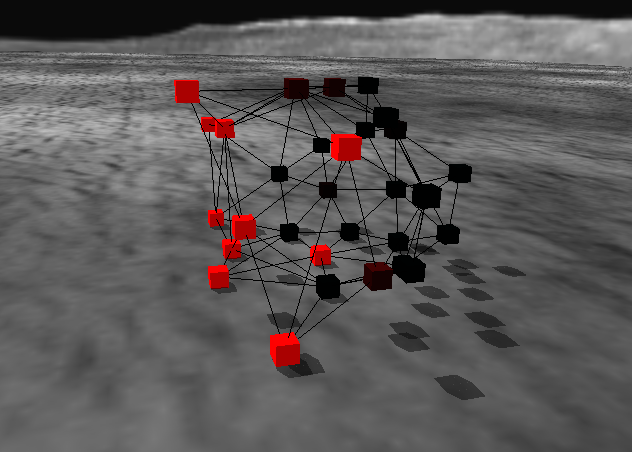#Wriggraph: A Kinetic Graph Model That Uniformly Describes Ontogeny and Motility of Artificial Creatures

Koji Sano1 and Hiroki Sayama1,2*
1 Department of Human Communication, University of Electro-Communications, Japan
2 Department of Bioengineering, Binghamton University, State University of New York
* Correspondent author (sayama at binghamton dot edu)

## Abstract

Earlier models of kinetic artificial creatures typically describe ontogenetic mechanisms and motion control mechanisms separately. The latter are often assumed free from morphological constraints, reducing the biological and physical plausibility of these creatures. To go over these limitations, here we propose Wriggraph, a kinetic graph model that describes both ontogeny and motility of graph-based artificial creatures in a unified framework. The utility of this model is demonstrated by constructing an artificial creature tetrad, which can spontaneously develop into a stable tetrahedral shape, move using differentiated nodes, home toward a predefined goal, and self-repair in events of node removal. All of these capabilities are achieved collectively by functionally identical nodes and their local interaction only. We expect a number of future extensions of this research project, including the automated acquisition of more complex morphologies and behaviors using evolutionary computation, and the revision of model assumptions to make node division mechanisms more realitsic.

## Papers

• Koji Sano and Hiroki Sayama, Wriggraph: A kinetic graph model that uniformly describes ontogeny and motility of artificial creatures, Artificial Life X: Proceedings of the Tenth International Conference on the Simulation and Synthesis of Living Systems, Bloomington, IN, 2006, MIT Press, pp.77-83. (PDF)
• 佐野浩司, 人工生命体の発生と運動を同一の枠組みで実現する動力学的グラフモデル, 電気通信大学大学院電気通信学研究科人間コミュニケーション学専攻修士論文, 2006. In Japanese. (PDF)

## Simulator

### Files

exe files(zip)

It includes simulator .exe file, Gene source file(It must be named source.txt), .x 3D object file and some .bmp texture files. You can change .x and texture files to modify simulator's screen. In that case, those files should be the same as former file name.

### Simulator Key & Mouse Controlls

 Left click and drag Rotation of the view point Left shift + Left click and drag Parallel translation of the view point C Set the regard point to the average of the position coordinate of all nodes Right shift + Left click and drag Rotation of the view point centering the regard point Space Remove all nodes, reread Gene file and set a new node F Forward camera B Backward camera H Halt movement of nodes and execution of Gene. Restart if you repush S + Left click a node Displaying "P" and "D" of the node. If you S + Left click where no node is, display goes out K + Left click a node Killing the node Forward key Rating up the count par 1 frame of execution of Gene, physical simulation and diffusion of "P" Backward key Rating down the count par 1 frame of execution of Gene, physical simulation and diffusion of "P"

### Samples

Here are some Gene samples. For more details, see thesis.

#### Simple division

Source:

``` PROG2 (
ADDPAR ( 0 1 )
GDIVIDE ( 0 )
)```

Image:#### Rod-like

Source:

``` PROG2 (
PROG3 (
< Increasing division factor >
TDIVIDE (
ADDPAR (
0
0.9
)
)
< Increasing anti-division factor >

ADDPAR (
0.2
MUL (
1 < Change this value! >
GETP ( 0 )
)
)
< Subtract anti-division factor from division factor >
SUBPAR (
0
MUL (
0.4
GETP ( 0.2 )
)
)
)
PROG3 (
< Increasing anti-division factor's "D" >
ADDDIF (
0.2
0.001
)
ELENGTH ( 0.2 )
SETCOLP ( 0 0.9 0.2 )
)
)```

Image:#### Roller

Source:

``` PROG3 (
PROG3 (
SETCOLP ( 0.2 0.4 0.4 )
ELENGTH ( 0.2 )
PROG3 (
DIFFRIC 0.6
DYNFRIC 0.6
SETP 0.6 0.5
)
)
< Division control code >
PROG3 (
GDIVIDE ( 0 )
IFT (
NOT GETP ( 0 )
ADDDIF ( 0 1 )
)
IFT (
LT ( GETD ( 0 ) 0.04 )
ADDPAR ( 0 1 )
)
)
< Motion control code >

PROG3 (
< 3. Comparing P[0.4] and value of SMELL after difffusion >
IFTE (
< 3.1.1 If P[0.4] is smaller than SMELL... >
< It means that the node's value of P[0.4] is bigger than neightbor nodes's one because of diffusion >
< so, the node is closer to goal object than the neighbor nodes >

LT GETP 0.4 SMELL
< 3.1.2 Shorten its edges >
SUBPAR 0.2 1
< 3.2.1 else... >
IFT LT GETP 0.2 2
< 3.2.2 lengthen its edges >
ADDPAR 0.2 1
)
SETD 0.4 1
< 1. Setting value of SMELL to P[0.4] >

SETP 0.4 SMELL
< 2. Diffusion occures >
)
)```

Image:#### Tetrad

Source:

``` PROG3 (
< Division control code >
PROG2 (
< simple division >
PROG3 (
IFT ( LT ( GETP ( 0.2 ) 0.0001 )
ADDPAR ( 0 0.09 ) )
SUBPAR ( 0 GETP ( 0.2 ) )
TDIVIDE ( 0 )
)
< managed division >
PROG3 (
SETD ( 0.2 0.1 )
ADDPAR ( SUBPAR ( 0.2 0.3 ) GETP ( 0.3 ) )
IFT ( LT ( GETP ( 0.3 ) 0.5 )
TDIVIDE ( 0.2 ) )
)
)
< Reaction-Diffusion System >

< activator: P[0.3] inhibitor: P[0.4] >
PROG2 (
PROG3 (
< Initializing values >
IFT ( NOT ( GETP ( 0.3 ) )
ADDPAR ( 0.3 0.1 ) )
IFT ( NOT ( GETP ( 0.4 ) )
ADDPAR ( 0.4 0.1 ) )
PROG3 (
SETD ( 0.3 0 )
SETD ( 0.4 0.25 )
IFT ( AND ( GROUND
AND ( GETP ( 1.0 )
LT ( GETP ( 1.0 )
0.5 ) ) )
ADDPAR ( 0.3 GETP ( 1.0 ) ) )
)
)
PROG2 (
< Reaction code >
ADDPAR ( 0.3 SUB ( MUL ( 0.9 GETP ( 0.3 ) )
MUL ( 0.6 GETP ( 0.4 ) ) ) )
ADDPAR ( 0.4 SUB ( MUL ( 0.7 GETP ( 0.3 ) )
MUL ( 0.3 GETP ( 0.4 ) ) ) )
)
)
< Motion control code >

PROG2 (
PROG3 (
< The node with high level activator can act as muscles >
ELENGTH ( ADDPAR ( 0.8 GETP ( 0.3 ) ) )
IFTE ( LT ( GETP ( 0.3 ) 0.5 )
SETP ( 0.8 0.5 )
PROG2 (
SETP ( 1.0 5 )
SETP ( 2 5 )
)
)
IMPULSE ( 0.8 0 )
)
< Direction of movement control code >
PROG2 (
IFT ( AND ( GROUND
AND ( GT ( GETP ( 0.4 ) 0.5 )
LT ( GETP ( 0.3 ) 0.5 ) ) )
PROG2 (
IFTE ( LT ( GETP ( 0.6 ) SMELL )
PROG2 (
SETP ( 1.0 0.9 )
SETP ( 2 0.9 )
)
PROG2 (
SETP ( 1.0 0.1 )
SETP ( 2 0.1 )
)
)
PROG2 (
SETP ( 0.6 SMELL )
SETD ( 0.6 0.5 )
)
)
)
PROG2 (
DYNFRIC ( 1.0 )
DIFFRIC ( 2 )
)
)
)
)```

Image: